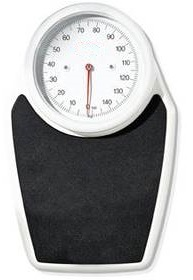﻿ Kilogram Converter/Calculator

# Kilogram Converter/CalculatorEnter value:

0 kilograms

Examples of Values to Enter:

100 micrograms
200 milligrams
10 centigrams
500 grams
25 pounds (or 25lb)
3 slugs

This kilogram calculator/converter converts the unit of weight entered in such as milligrams, grams, pounds, etc. into their equivalent kilograms value.

To use this calculator, a user enters the numerical value and the unit of the weight s/he wants to convert to kilograms. This can be in micrograms, milligrams, centigrams, grams, pounds, or slugs. The user then presses the 'Calculate' button, and the resultant value in unit kilograms is automatically computed and displayed.

Kilograms is a very important unit of measurement, because it is the SI unit of mass. Therefore, it is the most commonly used measurement of mass. It is used in practically every equation that has to do with mass, such as weight (W= mg), momentum (p=mv), centripetal force (F=mv2/r), and many, many other formulas. Being that kilogram is used in so many equations as the SI unit of mass, it is necessary to convert from other units to kilograms to calculate the equation. Since electronics encompasses so many different weight-bearing devices such as electronic scales, accelerometers, etc, working with kilograms is essential for making calculations.

### Examples

Convert 200,000 micrograms into kilograms.

200,000 micrograms= .0002 kg

Convert 5000 milligrams into kilograms.

5000 milligrams= .005 kg

Convert 5 minutes into kilograms.

500 grams= 0.5 kg

Convert 20 pounds into kilograms.

20 pounds= 9.07 kg

Convert 7 slugs into kilograms.

7 slugs= 102.16 kg

Related Resources